# Preface

This secton demonstrates applications of Picard's iteration scheme for power series determination of solutions to the initial value problems for second and higher order differential equations.

# Picard iterations

Picard's theorem on the existence and uniqueness of solutions of first order differential equations was discussed previously in the introductory section. Let us outline the main ideas in Picard's iteration procedure, starting with the first order differential equation
\begin{equation} \label{EqPicard.1} y' = f(x,y) , \qquad y \left( x_0 \right) = y_0 . \end{equation}
Here $$y' = {\text d}y/{\text d}x$$ is the derivative of function y(x). It is convenient to use the derivative operator $$\texttt{D} = {\text d}/{\text d}x ,$$ and rewrite Eq.\eqref{EqPicard.1} in operator form
$\texttt{D}y = f(x,y) , \qquad y \left( x_0 \right) = y_0 .$
The iteration procedure is very basic mathematical technique that conceptually sets the tone for a large class of analytical and numerical methods. We are going to apply this scheme for determination of series solutions for wide class of differential equations.

Since the differential equation contains the unbounded derivative operator, it is hard to expect that any iteration procedure applied to the differential equation \eqref{EqPicard.1} will lead to an acceptable solution. Therefore, the first step is to get rid of this unbounded operator by integration: \begin{equation} \label{EqPicard.2} y(x) = y\left( x_0 \right) + \int_{x_0}^x f\left( s,y(s) \right)\,{\text d} s . \end{equation} There are some advantages in converting the given initial value problem \eqref{EqPicard.1} into an equivalent problem without derivatives. You can recognize in the right-hand side integral the inverse to the derivative operator $$\texttt{D} = {\text d}/{\text d}x ,$$ acting in the space of smooth function vanishing at x0. So adding y(x0) is needed to satisfy the initial condition. Next, the form of Eq.\eqref{EqPicard.2} tells us that we can apply a fixed point theorem to the equation y = Φ(y) for a bounded integral operator $$\Phi (y) = \int_{x_0}^x f\left( s,y(s) \right)\,{\text d} s .$$ Eq.\eqref{EqPicard.2} is known as the Volterra integral equation of the second kind, which is suitable for the iteration procedure: \begin{equation} \label{EqPicard.3} \phi_{m+1} (x) = y\left( x_0 \right) + \int_{x_0}^x f\left( s,\phi_m (s) \right)\,{\text d} s , \qquad m=0,1,2,\ldots , \qquad \phi_0 = y\left( x_0 \right) . \end{equation} When the slope function f(x, y) is a Lipschitz continuous function with respect to variable y, the Picard's iteration \eqref{EqPicard.3} converges uniformly to a unique solution of the initial value problem \eqref{EqPicard.1} on some interval containing the initial point x0.

Picard's iteration scheme can be implemented in Mathematica in many ways. Some of them are presented below.

Clear[picardSeries, iterate];
iterate[initial_, flow_, psi_, n_, t_] :=
initial +
Expand@Apply[Subtract,
Assuming[{Subscript[t, n + 1] > 0},
Integrate[flow[Subscript[t, n + 1], psi], Subscript[t, n + 1]]] /. {{Subscript[t, n + 1] ->
Subscript[t, n]}, {Subscript[t, n + 1] -> 0}}]

The iteration step is called iterate, and it keeps track of the iteration order n so that it can assign a separate integration variable name at each step. As additional tricks to speed things up, I avoid the automatic simplifications for definite integrals by doing the integral as an indefinite one first, then using Subtract to apply the integration limits.

Instead of a generic simplification, I add Expand to the result in order to help the subsequent integration step recognize how to split up the integral over the current result. The last argument in iterate is the name that I intend to use as the base variable name for the integration, but it will get a subscript n attached to it.

picardSeries[initialVector_, flow_, n_, var_] :=
Module[{time},
Fold[iterate[initialVector, flow, #1, #2, time] &, initialVector,
Reverse[Range[n] - 1]] /. {Subscript[time, 0] -> var}]
Then iterate is called by picardSeries, using increasing subscripts starting with the outermost integration. This is implemented using Fold with a Reverse range of indices. The first input is the initial condition, followed by the function defining the flow (specifically, defined below as flow[t_, f_]). After the order n, I also specify the name of the independent variable var as the last argument. Here is the code from the following example involving the Riccati equation:
flow[t_, f_] := f^2 + t^2
picardSeries[1, flow, 3, x]

If you want intermediate results, too, here is a function that keeps them:

picardList[initialVector_, flow_, n_, var_] :=
Module[{time}, FoldList[
iterate[
initialVector, flow, #1, #2, time ] &,
initialVector, Reverse[Range[n] - 1]] /. {Subscript[time, _] -> var} ]
Then the previous code will be as follows
picardList[1, flow, 4, x] // TableForm

This solution works equally well for vector initial value problems, i.e., the flow can be a vector function and the initial condition a vector. It can be extended for higher order differential equations.

Example: Let us consider the initial value problem

$\texttt{D}y = x^2 + y^2 , \qquad y \left( 0 \right) = 1 .$
We convert this problem into the Volterra equation \eqref{EqPicard.2}, which leads to the Picard's iteration scheme:
$\phi_{n+1} (x) = 1 + \int_0^x \left( s^2 + \phi_n (s)^2 \right) {\text d} s = 1 + \frac{x^3}{3} + \int_0^x \phi_n^2 (s)\,{\text d} s , \qquad n=0,1,2,\ldots , \quad \phi_0 = 1.$
Application of Picard's iteration schemeyields a sequence of functions \begin{align*} \phi_1 (x) &= 1 + \frac{x^3}{3} + \int_0^x 1^2 {\text d} s = 1 + x + \frac{x^3}{3} , \\ \phi_2 (x) &= 1 + \frac{x^3}{3} + \int_0^x \phi_1^2 {\text d} s = 1 + x + x^2 + \frac{2\,x^3}{3} + \frac{x^4}{6} + \frac{2\,x^5}{15} + \frac{x^7}{63} , \\ \phi_3 (x) &= 1 + \frac{x^3}{3} + \int_0^x \phi_2^2 {\text d} s = 1 + x + x^2 + \frac{4\,x^3}{3} + \frac{5\,x^4}{6} + \frac{8\,x^5}{15} + \frac{29\,x^6}{90} + \frac{47\,x^7}{315} \\ & \quad + \frac{41\,x^8}{630} + \frac{299\,x^9}{11340} + \frac{4\,x^{10}}{525} + \frac{184\,x^{11}}{51975} + \frac{x^{12}}{2268} + \frac{4\,x^{13}}{12285} + \frac{x^{15}}{59535} . \end{align*} The next iteration requires utilization of a computer algebra system because its manual integration will take too much efforts. Of course, mathematica can handle it, but analytical expressions will grow as a snow ball. We compare the last approximation with the true expansion:
AsymptoticDSolveValue[{y'[x] == x^2 + (y[x])^2, y == 1}, y[x], {x, 0, 15}]
1 + x + x^2 + (4 x^3)/3 + (7 x^4)/6 + (6 x^5)/5 + (37 x^6)/30 + ( 404 x^7)/315 + (369 x^8)/280 + (428 x^9)/315 + (1961 x^10)/1400 + ( 75092 x^11)/51975 + (1238759 x^12)/831600 + (9884 x^13)/6435 + ( 17121817 x^14)/10810800 + (115860952 x^15)/70945875
$y = 1 + x + x^2 + \frac{4 x^3}{3} + \frac{7 x^4}{6} + \frac{6 x^5}{5} + \frac{37 x^6}{30} + \frac{404 x^7}{315} + \frac{369 x^8}{280} + \frac{428 x^9}{315} + \frac{1961 x^{10}}{1400} + \cdots .$
So we observe that the n-th iterative term gives a correct n-th degree polynomial accompanied by the noise terms of higher degree. With every iterative step, we obtain a better polynomial approximation that suffers by inaccurate higher degree noise polynomial. Now we compare Picard's approximation with the true solution.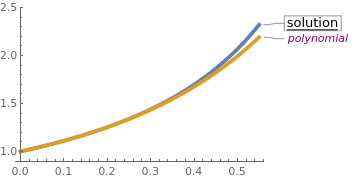sol = NDSolve[{y'[x] == x^2 + (y[x])^2, y == 1}, y[x], {x, 0, 0.8}]; phi[x_] = (1 + x + x^3/3)//N; For[k = 2, k <= 7, k++, phi[k][x_] = 1 + x^3/3 + Integrate[(phi[k - 1][s])^2, {s, 0, x}]] Do[Print["k= ", k, ", phi(k) = " phi[k][x]], {k, 2, 5}] f[x_] = phi[x]; Plot[{Callout[Evaluate[y[x] /. sol], "solution", Automatic, Frame -> True, LabelStyle -> Directive[Medium, Black, Underlined]], Callout[f[x], "polynomial", Automatic, LabelStyle -> Directive[Italic, Purple]]}, {x, 0, 0.55}, PlotRange -> {0.9, 2.5}, PlotStyle -> {Thickness[0.015]}] Third iterative polynomial approximation and the solution. Mathematica code

Using FoldList:
h = (y[#2][x_] = Function[{t}, 1 + Integrate[x^2 + #1^2, {x, 0, t}]][x]) &;
FoldList[h, 1, Range]
{1, 1 + x + x^3/3, 1 + x + x^2 + (2 x^3)/3 + x^4/6 + (2 x^5)/15 + x^7/63}
Another option is to use Function with NestList:    ■

Example: Let us consider the initial value problem

$\texttt{D}y = \left( 3 -x \right) y^3 , \qquad y \left( 0 \right) = 1 .$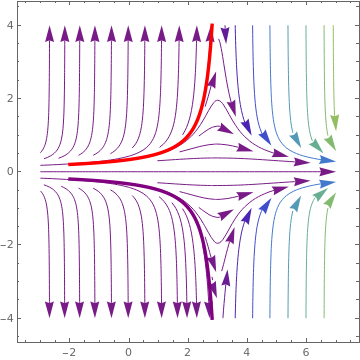field = StreamPlot[{1, (3 - x)*y^3}, {x, -3, 7}, {y, -5, 5}, StreamColorFunction -> "Rainbow", StreamPoints -> 70, StreamScale -> {Full, All, 0.04}]; s1 = NDSolve[{u'[x] == (3 - x)*(u[x])^3, u[-2] == 0.1999}, u[x], {x, -2, 3}]; sep1 = Plot[Evaluate[u[x] /. s1], {x, -2, 3}, PlotStyle -> {Red, Thickness[0.01]}, PlotRange -> {0, 4}]; s2 = NDSolve[{u'[x] == (3 - x)*(u[x])^3, u[-2] == -0.1999}, u[x], {x, -2, 3}]; sep2 = Plot[Evaluate[u[x] /. s2], {x, -2, 3}, PlotStyle -> {Purple, Thickness[0.01]}, PlotRange -> {-4, 0}]; Show[field, sep1, sep2] Direction field for the given Abel equation with two separatrix. Mathematica code

Substitution of the slope function $$f(x,y) = \left( 3 -x \right) y^3$$ into the Picard's scheme \eqref{EqPicard.3} yields $\phi_{m+1} (x) = 1 + \int_0^x \left( 3 -s \right) \phi_{m}^3 (s)\,{\text d} s , \qquad m=0,1,2,\ldots , \quad \phi_0 = 1 .$ Let us perform a few iterative steps:
\begin{align*} \phi_1 (x) &= 1 + \int_0^x \left( 3 -s \right) 1^3 {\text d} s = {\bf 1 + 3\,x} - \frac{x^2}{2} , \\ \phi_2 (x) &= 1 + \int_0^x \left( 3 -s \right) \phi_1^3 (s)\, {\text d} s = 1 + 3\,x + 13\,x^2 + \frac{45\, x^3}{2} + \frac{57\, x^4}{8} + \frac{45\, x^5}{4} + \frac{13\, x^6}{4} + \frac{3\, x^7}{8} + \frac{x^8}{64} , \\ \phi_3 (x) &= 1 + 3\,x + 13\,x^2 + 63\, x^3 + \frac{1839\, x^4}{8} + \frac{28197\, x^5}{40} + \frac{28399\, x^6}{16} + \frac{28149\, x^7}{8} + \frac{342967\, x^8}{64} + \cdots , \end{align*}
and so on. Every iteration contains some correct terms and noise terms of higher degree. This is expected because the given Abel equation is a variable coefficient equation.
AsymptoticDSolveValue[{y'[x] == (3 - x)*(y[x])^3, y == 1}, y[x], {x, 0, 10}]
1 + 3 x + 13 x^2 + 63 x^3 + 321 x^4 + 1683 x^5 + 8989 x^6 + 48639 x^7 + 265729 x^8 + 1462563 x^9 + 8097453 x^10
We can generate Picard's iterates with Mathematica
f[x_, y_] := (3 - x)*y^3;
yn[x_, n_, x0_, y0_] := If[n > 0, y0 + Integrate[f[s, yn[s, n - 1, x0, y0]], {s, x0, x}], y0]
Simplify[yn[x, 3, 0, 1]]
1 - (1/34078720)(-6 + x) x (17039360 + (-6 + x) x (-12779520 + (-6 + x) x (10649600 + (-6 + x) x (-6922240 + (-6 + x) x (3833856 + 5 (-6 + x) x (-359424 + (-6 + x) x (139776 + (-6 + x) x (-44928 + (-6 + x) x (11648 + (-6 + x) x (-2288 + (-6 + x) x (312 + (-6 + x) x (-26 + (-6 + x) x))))))))))))
Clear[h]
h = Function[{t}, 1 + Integrate[(3-x)* #^3, {x, 0, t}]][x] &;
NestList[h, 1, 3]
or
Clear[y, h]
h = Function[{t}, 1 + Integrate[(3-x)* #^3, {x, 0, t}]][x] &;
NestList[{1 + First@#, y[1 + First@#][x_] = h@Last@#} &, {0, 1}, 2]
y[x]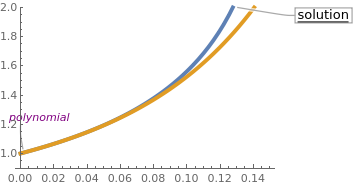sol = NDSolve[{y'[x] == (3 - x)*(y[x])^3, y == 1}, y[x], {x, 0, 0.15}] phi[x_] = 1 + 3*x - x^2/2; For[k = 2, k <= 4, k++, phi[k][x_] = 1 + Integrate[(3 - s)*(phi[k - 1][s])^3, {s, 0, x}]]; f[x_] = N[phi[x]] Plot[{Callout[Evaluate[y[x] /. sol], "solution", Automatic, Frame -> True, LabelStyle -> Directive[Medium, Black, Underlined]], Callout[f[x], "polynomial", Automatic, LabelStyle -> Directive[Italic, Purple]]}, {x, 0, 0.15}, PlotRange -> {0.9, 2}, PlotStyle -> {Thickness[0.015]}] Third iteration to the true solution. Mathematica code

■
Since every iteration step in \eqref{EqPicard.3} requires integration, it can be efficiently performed only when the slope function is a polynomial. Therefore, the general effectiveness of Picard’s method fails apparently even for relatively simple problems such as the pendulum equation. This criticisms can be overcomed by applying a well-known transformation technique or polynomial approximations (as shown by L.N. Trefethen using Chebyshev polynomials).

Second order differential equations

In this subsection, we specifically consider second order ordinary differential equations (ODEs for short), i.e., equations of the form
\begin{equation} \label{EqPicard.4} y'' = f(x,y,y' ) \qquad y\left( x_0 \right) = y_0 , \quad y' \left( x_0 \right) = y_1 . \end{equation}
The theory of second order ODEs is an extensive and highly developed part of mathematics, not least because of its numerous applications in the physical sciences. For example, Newton told us, with impressive foresight, that the radial motion of the space shuttle is governed by the equation
$\frac{{\text d}^2 r}{{\text d} t^2} = - \frac{M\,G}{r^2}$
where M is the mass of the earth and G is the gravitational constant. As another sample, one may consider a simple pendulum equation
$\ddot{\theta} + \omega^2 \sin \theta =0, \qquad \omega^2 = g/\ell .$
None of these equations is suitable to practical Picard's iteration because they involve nonpolynomial functions. Therefore, they can be solved with Picard's method only upon transformations into polynomial forms---it will be shown in the second part of the tutorial.

Integration twice the differential equation \eqref{EqPicard.4}, we obtain

$y(x) = y\left( x_0 \right) + \left( x- x_0 \right) y'\left( x_0 \right) + \int_{x_0}^x \left( x- t \right) f\left( t, y(t), y' (t) \right) {\text d} t.$
This equation is suitable for a fixed point theorem and the Picard's iteration yields
\begin{equation} \label{EqPicard.5} \phi_{m+1} (x) = y\left( x_0 \right) + \left( x- x_0 \right) y'\left( x_0 \right) + \int_{x_0}^x \left( x- t \right) f\left( t, \phi_m (t), \phi'_m (t) \right) {\text d} t , \qquad m= 0,1,2,\ldots , \qquad \phi_0 (x) = y\left( x_0 \right) + \left( x- x_0 \right) y'\left( x_0 \right) . \end{equation}
Sometimes (at least in case of linear equations) it is possible to integrate by parts and eliminate the derivative from the sign of the integral in Eq.\eqref{EqPicard.5}. Next, we illustrate the iteration procedure on several examples.

Example: Consider the case of the Airy equation that occurs in diffraction theory

$y'' + 8x\,y(x) = 0, \qquad y(0) = A, \quad y' (0) = B,$
with the initial conditions left undetermined. It is convenient to use some constants A and B that can be set to particular numerical values later. In particular, choosing A = 1, B = 0 and calling this case as "Dirichlet", and then A = 0, B = 1 and calling this case as "Neumann", we obtain two linearly independent solutions to the given Airy equation. The Picard's iteration scheme simply carries the unknown constants, A and B, through the calculation. Its solution is known and expressed through Airy functions:
$y(x) = A\,\frac{1}{2}\, \Gamma \left( \frac{2}{3} \right) \left[ 3^{1/3} \mbox{Ai}(-2x) + 3^{1/6} \mbox{Bi}(-2x) \right] + B\,\frac{\pi}{6\,\Gamma \left( \frac{2}{3} \right)} \left[ 3^{5/6} \mbox{Ai}(-2x) - 3^{1/3} \mbox{Bi}(-2x) \right] ,$
where $$\Gamma (2/3) = \int_0^{\infty} t^{-1/3} e^{-t} {\text d}t \approx 1.35412 ,$$ and Ai(x) and Bi(x) are the Airy function of the first kind and second kind, respectively; they can be defined by the improper Riemann integrals:
$\mbox{Ai}(x) = \frac{1}{\pi} \int_0^{\infty} \cos \left( \frac{t^3}{3} + xt \right) {\text d} t , \qquad \mbox{Bi}(x) = \frac{1}{\pi} \int_0^{\infty} \left[ \exp \left\{ - \frac{t^3}{3} + xt \right\} + \sin \left( \frac{t^3}{3} + xt \right) \right] {\text d} t .$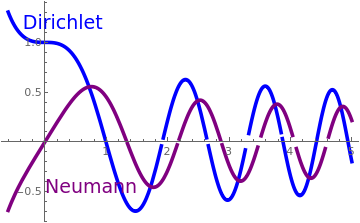s1 = DSolve[{y''[x] + 8*x*y[x] == 0, y == 1, y' == 0}, y[x], x] y1[x_] = 1/ 2 (3^(2/3) AiryAi[2 (-1)^(1/3) x] Gamma[2/3] + 3^(1/6) AiryBi[2 (-1)^(1/3) x] Gamma[2/3]); s2 = DSolve[{y''[x] + 8*x*y[x] == 0, y == 0, y' == 1}, y[x], x] y2[x_] = 1/ 12 (3 (-1)^(2/3) 3^(1/3) AiryAi[2 (-1)^(1/3) x] Gamma[1/3] - (-1)^( 2/3) 3^(5/6) AiryBi[2 (-1)^(1/3) x] Gamma[1/3]); plot1 = Plot[Evaluate[y[x] /. s1], {x, -0.6, 5}, PlotStyle -> {Blue, Thickness[0.01]}]; plot2 = Plot[Evaluate[y[x] /. s2], {x, -0.6, 5}, PlotRange -> {{-0.5, 5}, {-0.7, 1}}, PlotStyle -> {Purple, Thickness[0.01]}]; Show[plot1, plot2, Epilog -> {Inset[Text[Style["Dirichlet", Blue, 18]], {0.3, 1.2}], Inset[Text[Style["Neumann", Purple, 18]], {0.76, -0.45}]}] Or, using Callout, Plot[{Callout[y1[x], "Dirichlet", Above], Callout[y2[x], "Neumann", Right]}, {x, -0.5, 5}, PlotStyle -> {{Blue, Thickness[0.01]}, {Purple, Thickness[0.01]}}] Two solutions to the Airy equation with "Dirichlet" and "Neumann" initial conditions. Mathematica code

Mathematica knows its Maclaurin series expansion:
AsymptoticDSolveValue[{y''[x] + 8*x*y[x] == 0, y == aa, y' == bb}, y[x], {x, 0, 16}]
aa + bb x - (4 aa x^3)/3 - (2 bb x^4)/3 + (16 aa x^6)/45 + ( 8 bb x^7)/63 - (16 aa x^9)/405 - (32 bb x^10)/2835 + ( 32 aa x^12)/13365 + (64 bb x^13)/110565 - (128 aa x^15)/1403325 - ( 32 bb x^16)/1658475
Now we perform Picard's iterations. For the Airy equation with "Dirichlet" initial conditions (A = 1 and B = 0), the iteration scheme \eqref{EqPicard.5} takes the form
$\phi_{m+1} (x) = 1 - \int_0^x \left( x-t \right) 8t\,\phi_m (t) \,{\text d} t , \qquad m=0,1,2,\ldots , \quad \phi_0 = 1.$
Each term from the recursive scheme above satisfies the initial conditions $$\displaystyle \phi_m (0) = 1, \quad \phi'_m (0) = 0 .$$ Using Mathematica, we find first few terms:
\begin{align*} \phi_1 (x) &= 1 - \int_0^x \left( x-t \right) 8t \,{\text d} t = 1 - \frac{4\,x^3}{3} , \\ \phi_2 (x) &= 1 - \int_0^x \left( x-t \right) 8t \,\phi_1 (t) \,{\text d} t = 1 - \frac{4\,x^3}{3} + \frac{16}{45}\, x^6 , \\ \phi_3 (x) &= 1 - \int_0^x \left( x-t \right) 8t \,\phi_2 (t) \,{\text d} t = 1 - \frac{4\,x^3}{3} + \frac{16}{45}\, x^6 - \frac{16}{405}\, x^9 , \\ \phi_4 (x) &= 1 - \frac{4\,x^3}{3} + \frac{16}{45}\, x^6 - \frac{16}{405}\, x^9 + \frac{32}{13365}\, x^{12} , \end{align*}
and so on. As we see, each iteration brings use closer and closer to the twu polynomial expansion without noise terms. This is typical when the differential equation is homogeneous. When the equation is inhomogeneous, as in the previous example for the Riccati equation, noise terms are usually present.
phi1[x_] = 1 - Integrate[(x - t)*8*t, {t, 0, x}]
phi2[x_] = 1 - Integrate[(x - t)*8*t*phi1[t], {t, 0, x}]
phi3[x_] = 1 - Integrate[(x - t)*8*t*phi2[t], {t, 0, x}]
phi4[x_] = 1 - Integrate[(x - t)*8*t*phi3[t], {t, 0, x}]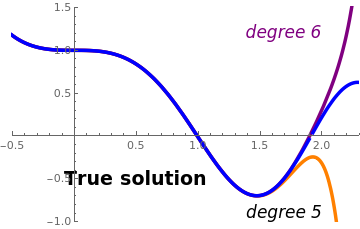txt = Graphics[{Black, Text[Style["True solution", Bold, 18], {0.5, -0.5}]}]; txt5 = Graphics[{Text[Style["degree 5", Italic, 16], {1.7, -0.9}]}]; txt6 = Graphics[{Text[Style["degree 6", Italic, Purple, 16], {1.7, 1.2}]}]; y1[x_] = 1/ 2 (3^(2/3) AiryAi[2 (-1)^(1/3) x] Gamma[2/3] + 3^(1/6) AiryBi[2 (-1)^(1/3) x] Gamma[2/3]); plot1 = Plot[Evaluate[y[x] /. s1], {x, -0.6, 5}, PlotStyle -> {Blue, Thickness[0.01]}]; phi5[x_] = 1 - (4 x^3)/3 + (16 x^6)/45 - (16 x^9)/405 + (32 x^12)/13365 - ( 128 x^15)/1403325; phi6[x_] =1 - (4 x^3)/3 + (16 x^6)/45 - (16 x^9)/405 + (32 x^12)/13365 - ( 128 x^15)/1403325 + (512 x^18)/214708725; pp5 = Plot[phi5[x], {x, -0.5, 2.3}, PlotStyle -> {Orange, Thickness[0.01]}, PlotRange -> {{-0.5, 2.3}, {-1, 1.5}}]; pp6 = Plot[phi6[x], {x, -0.5, 2.3}, PlotStyle -> {Purple, Thickness[0.01]}]; Show[pp5, pp6, plot1, txt, txt6, txt5] Comparison of true solution with two polynomial approximations. Mathematica code

Similarly, we obtain polynomial approximations for the initial conditions with A = 0 and B = 1 from the recursive formula
$\phi_{m+1} (x) = x - \int_0^x \left( x-t \right) 8t\,\phi_m (t) \,{\text d} t , \qquad m=0,1,2,\ldots , \quad \phi_0 = x.$
\begin{align*} \phi_1 (x) &= x - \int_0^x \left( x-t \right) 8t^2 \,{\text d} t = 1 - \frac{2\,x^4}{3} , \\ \phi_2 (x) &= x - \int_0^x \left( x-t \right) 8t \,\phi_1 (t) \,{\text d} t = 1 - \frac{2\,x^3}{3} + \frac{8}{63}\, x^7 , \\ \phi_3 (x) &= x - \int_0^x \left( x-t \right) 8t \,\phi_2 (t) \,{\text d} t = 1 - \frac{2\,x^3}{3} + \frac{8}{63}\, x^7 - \frac{32}{2835}\,x^{10} , \\ \phi_4 (x) &= x - \int_0^x \left( x-t \right) 8t \,\phi_3 (t) \,{\text d} t = 1 - \frac{2\,x^3}{3} + \frac{8}{63}\, x^7 - \frac{32}{2835}\,x^{10} + \frac{64\,x^{13}}{110 565} , \\ \phi_5 (x) &= 1 - \frac{2\,x^3}{3} + \frac{8}{63}\, x^7 - \frac{32}{2835}\,x^{10} + \frac{64\,x^{13}}{110 565} - \frac{32\,x^{16}}{1658475} , \\ \phi_6 (x) &= 1 - \frac{2\,x^3}{3} + \frac{8}{63}\, x^7 - \frac{32}{2835}\,x^{10} + \frac{64\,x^{13}}{110 565} - \frac{32\,x^{16}}{1658475} +\frac{128\, x^{19}}{283599225} . \end{align*}
■

Example: Consider the initial value problem

$y'' + 26\,y' + 25\, y = 0, \qquad y(0) = 2, \quad y' (0) = -26 .$
Since this is a constant coefficient homogeneous equation, the solution is known to be
$y(x) = e^{-x} + e^{-25\,x} = 2 - 26\, x + 313\,x^2 - \frac{7813}{3}\, x^3 + \frac{195 313}{12}\, x^4 + \frac{4882813}{60}\, x^5 - \frac{122070313}{360}\, x^6 - \cdots .$
Series[Exp[-x] + Exp[-25*x], {x, 0, 12}]
SeriesData[x, 0, {2, -26, 313, Rational[-7813, 3], Rational[195313, 12], Rational[-4882813, 60], Rational[122070313, 360], Rational[-3051757813, 2520], Rational[76293945313, 20160], Rational[-1907348632813, 181440], Rational[47683715820313, 1814400], Rational[-1192092895507813, 19958400], Rational[29802322387695313, 239500800]}, 0, 13, 1]
The Picard's ietration scheme \eqref{EqPicard.5} becomes
$\phi_{m+1} (x) = 2 -26\,x - \int_0^x \left( x-t \right) \left[ 26\,\phi'_m (t) + 25\,\phi_m (t) \right] {\text d} t , \qquad \phi_0 = 2 -26\,x.$
Upon integration by parts, we can modify this scheme:
$\phi_{m+1} (x) = 2 + 26\,x - \int_0^x \left[ 26 + 25 \left( x-t \right) \right] \phi_m (t) \, {\text d} t , \qquad \phi_0 = 2 -26\,x.$
First, we perform a few iterations using the standard scheme:
\begin{align*} \phi_1 (x) &= 2 - 26\,x - \int_0^x \left( x-t \right) \left[ -26*26 + 25*2 - 25*26*t \right] {\text d} t = 2 - 26\,x + 313\,x^2 + \frac{325}{3}\, x^3 , \\ \phi_2 (x) &= 2 - 26\,x - \int_0^x \left( x-t \right) \left[ 26\,\phi'_1 (t) + 25\,\phi_1 (t) \right] {\text d} t = 2 - 26\,x + 313\,x^2 + \frac{7813}{3}\, x^3 - \frac{5425}{4}\, x^4 - \frac{1625}{12}\, x^5 , \\ \phi_3 (x) &= 2 - 26\,x + 313\,x^2 + \frac{7813}{3}\, x^3 + \frac{195313}{12}\, x^4 + \frac{123695}{12}\, x^5 + \frac{123625}{72}\, x^6 + \frac{40625}{504}\, x^7 , \\ \phi_4 (x) &= 2 - 26\,x + 313\,x^2 + \frac{7813}{3}\, x^3 + \frac{195313}{12}\, x^4 - \frac{4882813}{60}\, x^5 - \frac{1397545}{24}\, x^6 - \frac{6306625}{504}\, x^7 - \frac{4146875}{4032}\, x^8 - \frac{1015625}{36288}\, x^9 . \end{align*}
phi0[x_] = 2 - 26*x;
phi1[x_] = 2 - 52*x - Integrate[(26+ 25*(x - t))*phi0[t] , {t, 0, x}]
phi2[x_] = 2 - 26*x - Integrate[(x - t)*(26*D[phi1[t], t] + 25*phi1[t]), {t, 0, x}]
phi3[x_] = 2 - 26*x - Integrate[(x - t)*(26*D[phi2[t], t] + 25*phi2[t]), {t, 0, x}]
phi4[x_] = 2 - 26*x - Integrate[(x - t)*(26*D[phi3[t], t] + 25*phi3[t]), {t, 0, x}]
Then we repeat calculations with the modified Picard's scheme:
\begin{align*} \phi_1 (x) &= 2 + 26\,x - \int_0^x \left[ 26+ 25 \left( x-t \right) \right] \phi_0 (t)\,{\text d} t = 2 - 26\,x + 313\,x^2 + \frac{325}{3}\, x^3 , \\ \phi_2 (x) &= 2 + 26\,x - \int_0^x \left[ 26+ 25 \left( x-t \right) \right] \phi_1 (t)\,{\text d} t = = 2 - 26\,x + 313\,x^2 + \frac{7813}{3}\, x^3 - \frac{5425}{4}\, x^4 - \frac{1625}{12}\, x^5 , \\ \phi_3 (x) &= 2 - 26\,x + 313\,x^2 + \frac{7813}{3}\, x^3 + \frac{195313}{12}\, x^4 + \frac{123695}{12}\, x^5 + \frac{123625}{72}\, x^6 + \frac{40625}{504}\, x^7 , \\ \phi_4 (x) &= 2 - 26\,x + 313\,x^2 + \frac{7813}{3}\, x^3 + \frac{195313}{12}\, x^4 - \frac{4882813}{60}\, x^5 - \frac{1397545}{24}\, x^6 - \frac{6306625}{504}\, x^7 - \frac{4146875}{4032}\, x^8 - \frac{1015625}{36288}\, x^9 . \end{align*}
phi0[x_] = 2 - 26*x;
phi1[x_] = 2 + 26*x - Integrate[(26 + 25*(x - t))*phi0[t], {t, 0, x}];
phi2[x_] = 2 + 26*x - Integrate[(26 + 25*(x - t))*phi1[t], {t, 0, x}];
phi3[x_] = 2 + 26*x - Integrate[(26 + 25*(x - t))*phi2[t], {t, 0, x}];
So we see that there is no difference which version to use---both provide the identical results.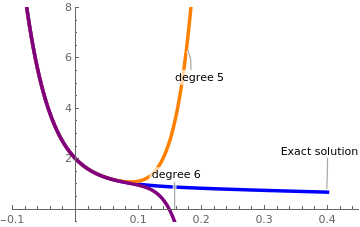phi5[x_] = 2 - 26 x + 313 x^2 - (7813 x^3)/3 + (195313 x^4)/12 - (4882813 x^5)/ 60 + (122070313 x^6)/360 + (133422575 x^7)/504 + (268788125 x^8)/ 4032 + (265484375 x^9)/36288 + (2890625 x^10)/8064 + (5078125 x^11)/ 798336; phi6[x_] = 2 - 26 x + 313 x^2 - (7813 x^3)/3 + (195313 x^4)/12 - (4882813 x^5)/ 60 + (122070313 x^6)/360 - (3051757813 x^7)/2520 - (64751405 x^8)/ 64 - (3441351875 x^9)/12096 - (908153125 x^10)/24192 - ( 2003828125 x^11)/798336 - (782421875 x^12)/9580032 - (9765625 x^13)/ 9580032; Plot[{Callout[Exp[-x] + Exp[-25*x], "Exact solution", Right], Callout[phi5[x], "degree 5", Right], Callout[phi6[x], "degree 6", Bottom]}, {x, -0.1, 0.4}, PlotRange -> {{-0.1, 0.45}, {-0.5, 8}}, PlotStyle -> {{Blue, Thickness[0.01]}, {Orange, Thickness[0.01]}, {Purple, Thickness[0.01]}}] Two polynomial approximations to the true solution. Mathematica code

■

Example: Consider the Legendre's differential equation

$\left( 1- x^2 \right) y'' -2x\,y' + \lambda\,y=0 \qquad\mbox{or}\qquad \frac{\text d}{{\text d}x} \left[ \left( 1 - x^2 \right) \frac{{\text d}y}{{\text d}x} \right] + \lambda \,y = 0 .$
Upon isolation of the second derivative and integration, we convert Legendre's equation to the following integro-differential equation
$y(x) = y(0) + x\, y' (0) + \int_0^x \left( x- t \right) \left[ t^2 y'' (t) + 2t\, y' (t) - \lambda\, y(t) \right] {\text d} t , \tag{A}$
where λ is a real parameter. The corresponding iteration procedure becomes
$\phi_{m+1} (x) = y(0) + x\, y' (0) + \int_0^x \left( x- t \right) \left[ t^2 \phi''_m (t) + 2t\, \phi'_m (t) - \lambda\, \phi_m (t) \right] {\text d} t , \qquad m=0,1,2,\ldots .$
Integration by parts of some terms in Eq.(A) leads to
$y(x) = y(0) + x\, y' (0) + \int_0^x \left[ \left( 4t -2x \right) y(t) - \lambda \left( x - t \right) y(t) + \left( 3t^2 - 2xt \right) y' (t) \right] {\text d} t . \tag{B}$
Since the right-hand side is a bounded operator, it is suitable for Picard's technique and we organize the iteration:
$\phi_{m+1} (x) = y(0) + x\, y' (0) + \int_0^x \left[ \left( 4t -2x \right) \phi_m (t) - \lambda \left( x - t \right) \phi_m (t) + \left( 3t^2 - 2xt \right) \phi'_m (t) \right] {\text d} t , \qquad m=0,1,2,\ldots , \quad \phi_0 = y(0) + x\, y' (0) .$
One more integration of Eq.(B) gives the relation
$\left( 1 - x^2 \right) y(x) = y(0) + x\, y' (0) + \int_0^x \left[ \left( 4t -2x -6t + 2x \right) y(t) - \lambda \left( x - t \right) y(t) \right] {\text d} t$
that is not suitable for Picard's iteration because it leads to non-polynomial integrands.

Now we perform a few iterations according to the algorithm (A). We split it into two parts: first we set y(0) = 1, y'(0) = 0, and then repeat with another initial conditions.

\begin{align*} \phi_1 (x) &= 1 - \int_0^x \left[ \left( x-t \right) \lambda \phi_0 (t) \right] {\text d} t = 1 - \lambda\,\frac{x^2}{2} , \\ \phi_2 (x) &= 1 + \int_0^x \left( x- t \right) \left[ t^2 \phi''_1 (t) + 2t\, \phi'_1 (t) - \lambda\, \phi_1 (t) \right] {\text d} t = 1 + \lambda \left( - \frac{x^2}{2} - \frac{x^4}{4} + \lambda\,\frac{x^4}{4} \right) , \\ \phi_3 (x) &= 1 - \frac{\lambda\, x^2}{720} \left[ 360 - 30 (-6 + \lambda) x^2 + (120 - 26 \lambda + \lambda^2) x^4 \right] , \\ \phi_4 (x) &= 1 - \lambda\,\frac{x^2}{2} - \lambda\,\frac{x^4}{4} + \lambda^2 \frac{x^4}{24} - \lambda\,\frac{x^6}{6} + 13 \lambda^2 \frac{x^6}{360} - \lambda^3\frac{x^6}{720} - \lambda \,\frac{x^8}{8} + 101 \lambda^2 \frac{x^8}{3360} - 17 \lambda^3 \frac{x^8}{10080} + \lambda^4 \frac{x^8}{40320} . \end{align*}
phi0[x_] = 1;
phi1[x_] = 1 + Integrate[-(x - t)*phi0[t]*lambda, {t, 0, x}]
phi2[x_] = 1+Integrate[(x - t)*( t^2 *D[phi1[t], t, t] + 2*t*D[phi1[t], t] - lambda*phi1[t]), {t, 0, x}]
phi3[x_] = 1 + Integrate[(x - t)*( t^2 *D[phi2[t], t, t] + 2*t*D[ph2[t], t] - lambda*phi2[t]), {t, 0, x}]
phi4[x_] = 1 + Integrate[(x - t)*( t^2 *D[phi3[t], t, t] + 2*t*D[ph3[t], t] - lambda*phi3[t]), {t, 0, x}]
We compare our answers with the true Maclaurin expansion
AsymptoticDSolveValue[{(1 - x^2)*y''[x] - 2*x*y'[x] + lambda*y[x] == 0, y == 1, y' == 0}, y[x], {x, 0, 7}]
1 - (lambda x^2)/2 + 1/12 (-3 lambda + lambda^2/2) x^4 + 1/30 (-3 lambda + lambda^2/2 + 2/3 (-3 lambda + lambda^2/2) - 1/12 lambda (-3 lambda + lambda^2/2)) x^6
$y = 1 - \lambda\,\frac{x^2}{2} - \lambda\,\frac{x^4}{4} + \lambda^2 \frac{x^4}{24} + \left( -\frac{\lambda}{6} + \frac{13\,\lambda^2}{360} - \frac{\lambda^3}{6!} \right) x^6 + \left( -\frac{\lambda}{8} + 101 \frac{\lambda^2}{3360} - 17 \frac{\lambda^3}{10080} + \frac{\lambda^4}{40320} \right) x^8 .$
Another linearly independent solution is obtained when we choose another initial approximation ϕ0 = x.
\begin{align*} \phi_1 (x) &= x + \int_0^x \left( x-t \right) \left[ 2*t - \lambda*t \right] {\text d} t = x - \lambda\,\frac{x^2}{2} , \\ \phi_2 (x) &= x + \int_0^x \left( x- t \right) \left[ t^2 \phi''_1 (t) + 2t\, \phi'_1 (t) - \lambda\, \phi_1 (t) \right] {\text d} t = x + \frac{x^3}{3} - \lambda \,\frac{x^3}{6} -\lambda\, \frac{x^4}{4} + \lambda^2 \frac{x^4}{24} , \\ \phi_3 (x) &= x + \frac{x^3}{720} \left[ 240 - 120 \lambda + 144 x^2 - 84 \lambda x^2 + 6 \lambda^2 x^2 - 120 \lambda x^3 + 26 \lambda^2 x^3 -\ lambda^3 x^3 \right] , \\ \phi_4 (x) &= x + \frac{x^3}{3} - \lambda\,\frac{x^3}{6} + \frac{x^5}{5} - \lambda\,\frac{7\,x^5}{60} + \lambda^2 \frac{x^5}{120} + \frac{x^7}{7} - \lambda\,\frac{37\,x^7}{420} + \lambda^2 \frac{11\,x^7}{1260} - \lambda^3 \frac{x^7}{5040} - \lambda\,\frac{x^8}{8} + \lambda^2 \frac{101\,x^8}{3360} - \lambda^3 \frac{17\,x^8}{10080} + \lambda^4 \frac{x^8}{40320} . \end{align*}
phi0[x_] = x;
phi1[x_] = x + Integrate[(x - t)*(t^2*D[phi0[t],t,t] + 2*t*D[phi0[t],t] -phi0[t]*lambda), {t, 0, x}]
phi2[x_] = x+Integrate[(x - t)*( t^2 *D[phi1[t], t, t] + 2*t*D[phi1[t], t] - lambda*phi1[t]), {t, 0, x}]
phi3[x_] = x + Integrate[(x - t)*( t^2 *D[phi2[t], t, t] + 2*t*D[ph2[t], t] - lambda*phi2[t]), {t, 0, x}]
phi4[x_] = x + Integrate[(x - t)*( t^2 *D[phi3[t], t, t] + 2*t*D[ph3[t], t] - lambda*phi3[t]), {t, 0, x}]
Its true Maclaurin expansion is
AsymptoticDSolveValue[{(1 - x^2)*y''[x] - 2*x*y'[x] + lambda*y[x] == 0, y == 0, y' == 1}, y[x], {x, 0, 8}]
x + 1/6 (2 - lambda) x^3 + 1/20 (4 - 2 lambda - 1/6 (2 - lambda) lambda) x^5 + 1/42 (4 - 2 lambda - 1/6 (2 - lambda) lambda + 1/2 (4 - 2 lambda - 1/6 (2 - lambda) lambda) - 1/20 lambda (4 - 2 lambda - 1/6 (2 - lambda) lambda)) x^7
$y = x + \frac{x^3}{3} - \lambda\,\frac{x^3}{6} + \left( \frac{1}{5} - \lambda\,\frac{7}{60} + \frac{\lambda^2}{120} \right) x^5 + \left( \frac{1}{7} - \lambda\,\frac{37}{420} + \lambda^2 \frac{11}{1260} - \frac{\lambda^3}{5040} \right) x^7 + \cdots .$
Now we use algorithm (B) and calculate
phi0[x_] = x;
phi1[x_] = x + Integrate[(4*t-2*x)*phi0[t] -(x - t)*lambda*phi0[t] + (3*t^2 - 2*x*t)*D[phi0[t],t], {t, 0, x}]
phi2[x_] = x + Integrate[(4*t-2*x)*phi1[t] -(x - t)*lambda*phi1[t] + (3*t^2 - 2*x*t)*D[phi1[t],t], {t, 0, x}]
phi3[x_] = x + Integrate[(4*t-2*x)*phi2[t] -(x - t)*lambda*phi2[t] + (3*t^2 - 2*x*t)*D[phi2[t],t], {t, 0, x}]
phi4[x_] = x + Integrate[(4*t-2*x)*phi3[t] -(x - t)*lambda*phi3[t] + (3*t^2 - 2*x*t)*D[phi3[t],t], {t, 0, x}]
So we see that algorithms (A) and (B) are equivalent.    ■

Example: Consider the initial value problem for the Lane--Emden equation

$\frac{{\text d}^2 y}{{\text d}t^2} + \frac{2}{t}\,\frac{{\text d}y}{{\text d}t} + y^5 =0 , \qquad y(0) = 1.$
This second order differential equation is named after the American astrophysicist Jonathan Homer Lane (1819--1880) and the Swiss astrophysicist and meteorologist Jacob Robert Emden (1862--1940). It is a so called the singular differential equation having a critical point at t = 0. The solutions of the Lane--Emden equation which are finite at the origin have necessarily dy/dt = 0 at t = 0. The Lane--Emden operator admits a factorizations:
$t^{-2} \frac{\text d}{{\text d}t} \left( t^2 \frac{{\text d}y}{{\text d}t} \right) + y^n =0 \qquad\mbox{or} \qquad t^{-1} \frac{{\text d}^2}{{\text d}t^2} \left( t\, y(t) \right) + y^n =0 ,$
where the homogeneous Lane--Emden equation is known to have an explicit solution for n = 0, 1, 5. For his equation, we don't have uniqueness and existence theorems, and there could be singular solutions. In particular, an explicit solution for n = 5 is
$y = \psi (t) = \left( 1 + \frac{t^2}{3} \right)^{-1/2} = 1 - \frac{t^2}{6} + \frac{t^4}{24} - \frac{5\,t^6}{432} + \frac{35}{10 368}\,t^8 - \frac{7\, t^{10}}{6912} + \cdots .$
The initial approximation is y0 = 1 follows from the initial condition. To find the next iterative term, we need to invert the Lane---Emden operator:
$L \left[ y \right] \equiv t^{-2} \frac{\text d}{{\text d}t} \left( t^2 \frac{{\text d}y}{{\text d}t} \right) = f(t) \qquad \Longrightarrow \qquad L^{-1} \left[ f \right] = y(0) + \int_0^t t^{-2} {\text d}t \int_0^t s^2 f(s)\,{\text d}s = y(0) + \int_0^t \frac{s}{t} \left( t-s \right) f(s) \,{\text d}s .$
Then we find the next iterative term:
$y_1 (t) = 1 - \int_0^t \frac{s}{t} \left( t-s \right) {\text d}s = 1 - \frac{t^2}{6} .$
Integrate[s/t*(t - s), {s, 0, t}]
The second one becomes
$y_2 (t) = 1 - \int_0^t \frac{s}{t} \left( t-s \right) \left( 1 - \frac{s^2}{6} \right)^5 {\text d}s = 1 - \frac{t^2}{6} + \frac{t^4}{24} - \frac{5\,t^6}{756} + \frac{5\,t^8}{7776} - \frac{t^{10}}{28 512} + \frac{t^{12}}{1 213 056} .$
Integrate[s/t*(t - s)*(1 - s^2 /6)^5 , {s, 0, t}]
We iterate next
$y_3 (t) = 1 - \int_0^t \frac{s}{t} \left( t-s \right) \left( 1 - \frac{s^2}{6} + \frac{s^4}{24} - \cdots \right)^5 {\text d}s = 1 - \frac{t^2}{6} + \frac{t^4}{24} - \frac{5\,t^6}{432} + \cdots .$
f2[t_] = 1 - t^2/6 + t^4/24 - (5 t^6)/756 + (5 t^8)/7776 - t^10/ 28512 + t^12/1213056;
Integrate[s/t*(t - s)*(f2[s])^5, {s, 0, t}]
where ··· denote the noise terms of order O(t8. So with three iterations, we obtaine an accurate 6-degree polynomial approximation of the actual solution.    ■

N-th order differential equations

Consider a n-th order (nonlinear) differential equation subject to the initial conditions:
\begin{equation} \label{EqPicard.n1} y^{(n)} = f(x,y,y' , \ldots , y^{(n-1)} ) , \qquad y\left( x_0 \right) = \alpha_0 , \quad y'\left( x_0 \right) = \alpha_1 , \quad \ldots , \quad .y^{(n-1)}\left( x_0 \right) = \alpha_{n-1} . \end{equation}
As usual, $$y^{(k)} = \texttt{D}^k y = {\text d}^k / {\text d} x^k$$ is the k-th derivative of the function y(x), with $$y' = \texttt{D}\, y = {\text d} / {\text d} x$$ and $$y'' = \texttt{D}^2 y = {\text d}^2 / {\text d} x^2 .$$ It is assumed that the given initial value problem \eqref{EqPicard.n1} has a unique solution for given n constants α0, α1, … , αn-1.

We integrate Eq.\eqref{EqPicard.n1} repeatedly, n times, to get

\begin{equation} \label{EqPicard.n2} y(x) = \frac{1}{(n-1)!} \int_{x_0}^x \left( x- t \right)^{n-1} f(t)\,{\text d}t + \sum_{k=0}^{n-1} \frac{\alpha_k}{k!}\, x^k . \end{equation}
Here
$f(t) = F\left( t, y(t), y' (t), \ldots , y^{(n-1)} (t) \right)$
and we call
$p(t) = \alpha_0 + \alpha_1 t + \frac{\alpha_2}{2!}\, t^2 + \cdots + \frac{\alpha_{n-1}}{(n-1)!}\, t^{n-1}$
the initial polynomial because it satisfies the given initial conditions in \eqref{EqPicard.n1}. Equation \eqref{EqPicard.n2} is solved now by iteration, upon choosing a suitable starting function
$\phi_0 = p_0 (t)$
that satisfies the initial conditions and then substituting φ0 into the right hand side of \eqref{EqPicard.n1}, i.e. \eqref{EqPicard.n2}, which can be integrated to yield ϕ1. Repeating the iterative process, we get the general formula to obtain ϕm+1 from ϕm
\begin{equation} \label{EqPicard.n3} \phi_{m+1}(x) = \frac{1}{(n-1)!} \int_{x_0}^x \left( x- t \right)^{n-1} f(\phi_m (t))\,{\text d}t + \sum_{k=0}^{n-1} \frac{\alpha_k}{k!}\, x^k , \qquad m = 0,1,2, \ldots . \end{equation}
The iteration process \eqref{EqPicard.n3} is terminated when the required degree of accuracy is attained.

Example: The Blasius equation of boundary layer flow is a third-order nonlinear differential equation:

$2\,f''' + f\,f'' = 0, \qquad f(0) = f' (0) =0, \quad f'' (0) = b ,$
where
$b \approx 0.332057336215196298937180062010$
is the Blasius constant that assures the behavior of the first derivative at infinity: $$\lim_{\eta \to \infty} f' (\eta ) = 1.$$ This constant was calculated by V.P.Varin in 2013. Now we convert the initial value problem for the Blasius equation into the integral equation
$y(x) = b\,\frac{x^2}{2} - \frac{1}{2} \int_0^x \frac{\left( x - s \right)^2}{2!} \,f(s)\,f'' (s)\,{\text d}s . \tag{A}$
Integration by parts yields
$y(x) = b\,\frac{x^2}{2} + \frac{1}{2} \int_0^x f' (s) \left[ f'(s) \, \frac{(x-s)^2}{2} - f(s) \,(x-s) \right] {\text d}s . \tag{B}$
So we have two algorithms to perform Picard's iteration. According to these algorithms, we have two recursive calls:
\begin{align*} \phi_{m+1} (x) &= b\,\frac{x^2}{2} - \frac{1}{2} \int_0^x \frac{\left( x - s \right)^2}{2!} \,\phi_m (s)\,\phi''_m (s)\,{\text d}s , \tag{A1} \\ \phi_{m+1} (x) &= b\,\frac{x^2}{2} + \frac{1}{2} \int_0^x \phi'_m (s) \left[ \phi'_m (s) \, \frac{(x-s)^2}{2} - \phi_{m}(s) \,(x-s) \right] {\text d}s . \tag{B1} \end{align*}
Now we make a numerical experiment and use each of these two algorithms to generate polynomial approximations. According to algorithm A, we have
Clear[b]; phi0[x_] = b*x^2 /2;
phi1[x_] = phi0[x] - (1/2)* Integrate[(x - s)^2 /2 *phi0[s]*D[phi0[s], s, s], {s, 0, x}]
phi2[x_] = phi0[x] - (1/2)* Integrate[(x - s)^2 /2 *phi1[s]*D[phi1[s], s, s], {s, 0, x}]
phi3[x_] = phi0[x] - (1/2)* Integrate[(x - s)^2 /2 *phi2[s]*D[phi2[s], s, s], {s, 0, x}]
phi4[x_] = phi0[x] - (1/2)* Integrate[(x - s)^2 /2 *phi3[s]*D[phi3[s], s, s], {s, 0, x}];
(b x^2)/2 + 1/2 (-(1/120) b^2 x^5 + (11 b^3 x^8)/80640 - (5 b^4 x^11)/2128896 + ( 9299 b^5 x^14)/232475443200 - (2173649 b^6 x^17)/ 5690998849536000 + (13722337 b^7 x^20)/3707279250554880000 - ( 27184438601 b^8 x^23)/827264535644319252480000 + ( 12320831753849 b^9 x^26)/51621307024205521354752000000 - ( 26703222167681 b^10 x^29)/17411469772287413079716659200000 + ( 8839351203863 b^11 x^32)/1134461610122109279151325184000000 - ( 8358051664337 b^12 x^35)/269457504613882447937132298240000000 + ( 2470778586557 b^13 x^38)/ 25469395057957561129385412526080000000 - (6001593809 b^14 x^41)/ 28647246829058138762679995596800000000 + (36769 b^15 x^44)/ 142746186329541437494206136320000000 - (b^16 x^47)/ 7588617146791990762049372160000000)
\begin{align*} \phi_{1} (x) &= b\,\frac{x^2}{2} - \frac{1}{2} \int_0^x \frac{\left( x - s \right)^2}{2!} \,b\,\frac{s^2}{2}\, b\,{\text d}s = b\,\frac{x^2}{2} - \frac{b^2 x^5}{240} , \\ \phi_{2} (x) &= b\,\frac{x^2}{2} - \frac{b^2 x^5}{240} + \frac{1}{2}\cdot \frac{11\,b^3 x^8}{80640} - \frac{1}{2}\cdot \frac{b^4 x^{11}}{2851200} , \\ \phi_{3} (x) &= b\,\frac{x^2}{2} - \frac{b^2 x^5}{240} + \frac{1}{2}\cdot \frac{11\,b^3 x^8}{80640} - \frac{1}{2}\cdot \frac{5\,b^4 x^{11}}{2128896} + \frac{1}{2}\cdot \frac{10033 \,b^5 x^{14}}{697426329600} - \frac{1}{2}\cdot \frac{5449\, b^6 x^{17}}{62538448896000} + \frac{1}{2}\cdot \frac{83\, b^7 x^{20}}{285937827840000} - \frac{1}{2}\cdot \frac{b^8 x^{23}}{3141177532416000} , \\ \phi_{4} (x) &= b\,\frac{x^2}{2} - \frac{b^2 x^5}{240} + \frac{1}{2}\cdot \frac{11\,b^3 x^8}{80640} - \frac{1}{2}\cdot \frac{5\,b^4 x^{11}}{2128896} + \frac{1}{2}\cdot \frac{9299 \,b^5 x^{14}}{232475443200} - \frac{1}{2}\cdot \frac{2173649\, b^6 x^{17}}{5690998849536000} + \cdots , \end{align*}
and so on. Now we repeat similar iterations based on algorithm (B):
psi1[x_] = phi0[x] + (1/2)* Integrate[ D[phi0[s], s]*((x - s)^2 /2 *D[phi0[s], s] - (x - s)*phi0[s]), {s, 0, x}];
psi2[x_] = phi0[x] + (1/2)* Integrate[ D[psi1[s], s]*((x - s)^2 /2 *D[psi1[s], s] - (x - s)*psi1[s]), {s, 0, x}];
psi3[x_] = phi0[x] + (1/2)* Integrate[ D[psi2[s], s]*((x - s)^2 /2 *D[psi2[s], s] - (x - s)*psi2[s]), {s, 0, x}]
(b x^2)/2 + 1/2 (-(1/120) b^2 x^5 + (11 b^3 x^8)/80640 - (5 b^4 x^11)/2128896 + ( 10033 b^5 x^14)/697426329600 - (5449 b^6 x^17)/62538448896000 + ( 83 b^7 x^20)/285937827840000 - (b^8 x^23)/3141177532416000)
\begin{align*} \psi_{1} (x) &= b\,\frac{x^2}{2} + \frac{1}{2} \int_0^x b\,s \left[ \frac{\left( x - s \right)^2}{2!} \,bs - b\,\frac{s^2}{2} \left( x - s \right) \right] {\text d}s = b\,\frac{x^2}{2} - \frac{b^2 x^5}{240} , \\ \psi_{2} (x) &= b\,\frac{x^2}{2} - \frac{b^2 x^5}{240} + \frac{1}{2}\cdot \frac{11\,b^3 x^8}{80640} - \frac{1}{2}\cdot \frac{b^4 x^{11}}{2851200} , \\ \psi_{3} (x) &= b\,\frac{x^2}{2} - \frac{b^2 x^5}{240} + \frac{1}{2}\cdot \frac{11\,b^3 x^8}{80640} - - \frac{1}{2}\cdot \frac{5\,b^4 x^{11}}{2128896} + \frac{1}{2}\cdot \frac{10033 \,b^5 x^{14}}{697426329600} - \frac{1}{2}\cdot \frac{5449\, b^6 x^{17}}{62538448896000} + \cdots . \end{align*}
Therefore, we conclude that both algorithms give the identical outputs. Now we compare Picard's polynomial approximations with true Maclaurin expansion.
AsymptoticDSolveValue[{f'''[x] + (1/2)*f[x]*f''[x] == 0, f == 0, f' == 0, f'' == b}, f[x], {x, 0, 22}]
(b x^2)/2 - (b^2 x^5)/240 + (11 b^3 x^8)/161280 - ( 5 b^4 x^11)/4257792 + (9299 b^5 x^14)/464950886400 - ( 1272379 b^6 x^17)/3793999233024000 + ( 19241647 b^7 x^20)/3460127300517888000
$f(x ) = b\,\frac{x^2}{2} - \frac{b^2 x^5}{240} + \frac{11\,b^3 x^8}{161280} - \frac{5\,b^4 x^{11}}{4257792} + \frac{9299 \,b^5 x^{14}}{464950886400} - \frac{1272379\, b^6 x^{17}}{3793999233024000} + \frac{19241647\, b^7 x^{20}}{3460127300517888000} - \cdots .$
In fact, a reasonable pattern can be established when extending the series above:
$f(x ) = \sum_{n\ge 0} \left( -\frac{1}{2} \right) \frac{b^{n+1} C_n}{(3n+2)!}\, x^{3n+2} ,$
where constants Cn are determined through the integrations.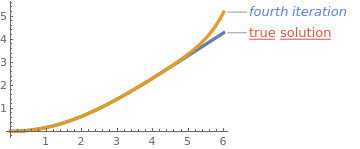Fourth Picard's iteration gives a good approximation to the solution of the Blasius equation on the interval {0,3]. Plot[{Callout[Evaluate[f[x] /. blasius], "true solution", Automatic, LabelStyle -> Directive[Underlined, Medium, ColorData[106, 3]]], Callout[phi4[x] /. b -> 0.332057336, "fourth iteration", Automatic, LabelStyle -> Directive[Italic, Medium, ColorData[106, 1]]]}, {x, 0, 6}, PlotStyle -> Thickness[0.015]] Fourth Picard;s approximation and the solution to the Blasius equation. Mathematica code

■

1. Borisut, P., Khammahawong, K. and Kumam, P., Fixed Point Theory Approach to Existence of Solutions with Differential Equations, 2018.
2. Fabrey, T., Picard’s Theorem, The American Mathematical Monthly, 1964, Vol. 79, Issue 9, pp. 1020--1023. https://doi.org/10.1080/00029890.1972.11993177
3. Brouwer fixed-point theorem, Wikipedia.
4. Grigorieva, E., Methods of Solving Sequence and Series Problems, Birkhäuser; 1st ed. 2016.
5. Islam, M.S., Fixed point technique in differential equations, Dissertation, International Centre for Theoretical Physics, P.O. Box 586, 1-34100 Tneste, Italy, 1997.
6. Pata, V., Fixed Point Theorems and Applications, Milan, Italy.
7. Robin, W.A., Solving differential equations using modified Picard iteration, International Journal of Mathematical Education in Science and Technology, 2010, Volume 41, Issue 5, pp. 649--665. https://doi.org/10.1080/00207391003675182
8. Sochacki, J., and Parker, E.G., The Modified Picard (Power Series Method).
9. Trefethen, L.N., Birkisson, A., Driscoll, T.A., Exploring ODEs, SIAM-Society for Industrial and Applied Mathematics (December 21, 2017), ISBN-13 : 978-1611975154
10. Varin, V.P., A solution of the Blasius problem, Keldysh Institute, Preprint No. 40, 2013.
11. Yu, Lien-Tsai and Chen, Cha'o-Kuang, The solution of the Blasius equation by the differential transformation method, Mathematical and Computer Modelling, 1998, Volume 28, Issue 1, July 1998, Pages 101-111. https://doi.org/10.1016/S0895-7177(98)00085-5